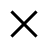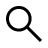Buscar

# Best sites for solving equationsWho I am
Martí Micolau
@martímicolau

Best sites for solving equations. Here is a simple guide on which sites to consider to solve all the problems of equations but also of algebra, math problems, logarithms and much more.

On the web there are many solutions to solve math homework and also for solve inequalities and equations.

## What is an equation

But what is an equation? It is nothing more than a mathematical equality between two expressions, called members, containing one or more variables, called unknowns, and constants (numbers), verified for particular values ​​of the unknowns.

The set of solutions is represented by those values ​​which, inserted in place of the unknowns, lead to equality.

## Best sites to solve equations onlineHere is a list of the most relevant equation solving sites to help you in a short time to overcome complex mathematical problems, showing you all those steps that are useful to both teachers and students and then check the final result.

### 1. YouMathYouMath is a real one-click online equation solver. It solves equations of any degree, divided, logarithmic, exponential, trigonometric and so on.

Please pay attention to the use of parentheses and if the equation contains fractions, logarithms or roots check the syntax carefully.

YouMath not only provides solutions of the equation but also a graphical representation of the solutions, useful in the case of equations with two unknowns. With three or more unknowns, the tool reports the sets of solutions in algebraic form.

### 2. Mini Math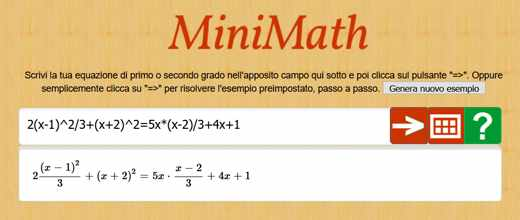MiniMath is equation calculator on a multilingual site where you can solve equations online. All you have to do is write your first or second degree equation in the indicated field and click on the “=>” button. Alternatively you can view all the examples proposed.

MiniMath is also available as an Android app and solves not only equations but also expressions.

### 3. Math PowerMathPower is a math help solver website where you can enter your equation quickly and press the button Solve to get the result. But the platform not only solves the exercises you enter, but also provides detailed and prepared explanations after giving you the solution.

### 4. The empire of Numbers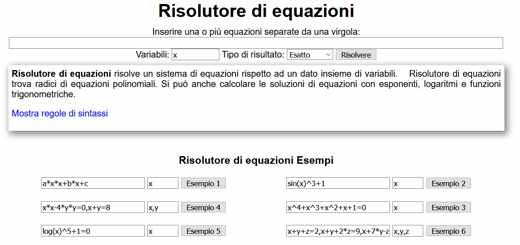Empire of Numbers - Equation Solver offers an equation solver capable of solving a system of equations with respect to a given set of variables. Find roots of polynomial equations and calculate solutions of equations with logarithms, exponents and trigonometric functions.

To use it you must enter one or more equations separated by a comma, specifying Variables e Result type (Exact or Numeric) and then press the button Solve.

In the lower part, the syntax rules are explained and examples of solving equations are provided.

### 5. MathematicaSchool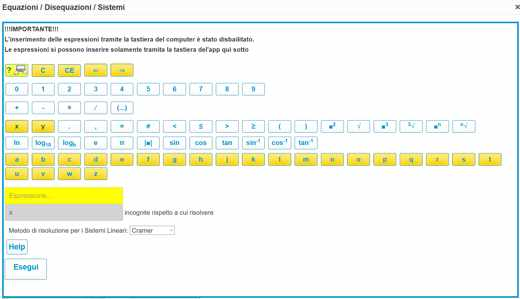MathematicaSchool is an online equation solving calculator aimed at students doing math exercises. Also available for Android devices, it is able to guarantee the resolution of expressions, equations, inequalities and systems.

For each operation, all the individual steps are shown and symbolic calculation is used.

Pigging on your pulse Equations / Inequalities / Systems you can access a real tool where you can enter your equation using only the program keyboard. To get the result of the equation typed, just press the button Run.

### 6. Wolfram | AlphaWolfram|Aplha is a computational knowledge engine that interprets the keyword entered by the user and proposes an answer. It offers an effective and easy-to-use tool for solving equations. All you have to do is type the equation in the appropriate field and press the button Submit and solve second degree equations.

## How to solve phrases with photos

In addition to the sites, there are also apps for Android and iOS that allow you to solve expressions with photos with modern tools. Here are the most effective ones:

Math Scanner (Android, iOS), it's a smart calculator app. It helps to solve the equations just by entering the formula. Just point the camera at a problem and the photo calculator will magically display the result with detailed step-by-step instructions.

Photomath (Android, iOS), with more than 100 million downloads, this app allows you to scan the problem with printed and handwritten text. Taking advantage of your device's camera you will have to enter the equation and the app's scientific calculator will do the rest by answering your questions.

Microsoft Math Solver (Android, iOS), provides assistance with arithmetic, algebra, calculus, statistics and so on. It is based on technological intelligence and by using your camera to take the picture of an equation, the app will immediately recognize the problem providing the desired solution.

FastMath (Android, iOS), after framing the mathematical equation to be solved with your camera, the app will immediately perform the required calculation. You can download it for free but to take advantage of all its functions you will have to subscribe to a subscription of 10,99 euros per month.

## Other useful articles

• Best sites to do the logical and grammatical analysis of a sentence
• App to translate
• Best Latin Translators

### Related Items

##### add a comment of Best sites for solving equations
Comment sent successfully! We will review it in the next few hours.